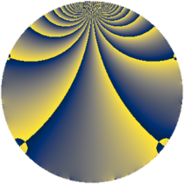# Properties

 Label 1183.2.qLevel $1183$ Weight $2$ Character orbit 1183.q Rep. character $\chi_{1183}(316,\cdot)$ Character field $\Q(\zeta_{6})$ Dimension $156$ Sturm bound $242$

# Related objects

## Defining parameters

 Level: $$N$$ $$=$$ $$1183 = 7 \cdot 13^{2}$$ Weight: $$k$$ $$=$$ $$2$$ Character orbit: $$[\chi]$$ $$=$$ 1183.q (of order $$6$$ and degree $$2$$) Character conductor: $$\operatorname{cond}(\chi)$$ $$=$$ $$13$$ Character field: $$\Q(\zeta_{6})$$ Sturm bound: $$242$$

## Dimensions

The following table gives the dimensions of various subspaces of $$M_{2}(1183, [\chi])$$.

Total New Old
Modular forms 272 156 116
Cusp forms 216 156 60
Eisenstein series 56 0 56

## Trace form

 $$156q + 80q^{4} + 18q^{6} - 80q^{9} + O(q^{10})$$ $$156q + 80q^{4} + 18q^{6} - 80q^{9} - 20q^{10} - 6q^{11} + 20q^{12} + 8q^{14} - 6q^{15} - 68q^{16} + 4q^{17} + 12q^{20} + 2q^{22} + 10q^{23} - 12q^{24} - 144q^{25} - 12q^{27} - 6q^{29} - 16q^{30} - 36q^{32} + 30q^{33} - 4q^{35} + 94q^{36} + 42q^{37} - 4q^{38} - 140q^{40} - 30q^{41} - 4q^{42} - 20q^{43} - 12q^{46} + 18q^{48} + 78q^{49} + 18q^{50} - 52q^{51} + 40q^{53} - 12q^{54} + 22q^{55} + 12q^{56} + 12q^{58} - 18q^{59} - 14q^{61} - 8q^{62} - 12q^{63} - 60q^{64} + 4q^{66} + 24q^{67} + 20q^{68} - 20q^{69} + 24q^{71} - 60q^{72} + 22q^{74} - 62q^{75} + 18q^{76} - 8q^{77} + 36q^{79} + 72q^{80} - 70q^{81} - 42q^{82} - 18q^{84} + 48q^{85} + 10q^{87} - 34q^{88} + 12q^{89} + 72q^{90} - 64q^{92} + 18q^{93} + 36q^{94} + 12q^{95} - 6q^{97} + O(q^{100})$$

## Decomposition of $$S_{2}^{\mathrm{new}}(1183, [\chi])$$ into newform subspaces

The newforms in this space have not yet been added to the LMFDB.

## Decomposition of $$S_{2}^{\mathrm{old}}(1183, [\chi])$$ into lower level spaces

$$S_{2}^{\mathrm{old}}(1183, [\chi]) \cong$$ $$S_{2}^{\mathrm{new}}(13, [\chi])$$$$^{\oplus 4}$$$$\oplus$$$$S_{2}^{\mathrm{new}}(91, [\chi])$$$$^{\oplus 2}$$$$\oplus$$$$S_{2}^{\mathrm{new}}(169, [\chi])$$$$^{\oplus 2}$$